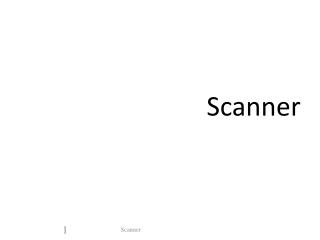DownloadDownload PresentationScanner

# Scanner

Download Presentation## Scanner

- - - - - - - - - - - - - - - - - - - - - - - - - - - E N D - - - - - - - - - - - - - - - - - - - - - - - - - - -
##### Presentation Transcript

1. Scanner

2. Introduction • A scanner, sometimes called a lexical analyzer • A scanner : • gets a stream of characters (source program) • divides it into tokens • Tokens are units that are meaningful in the source language. • Lexemes are strings which match the patterns of tokens.

3. Examples of Tokens in C

4. Scanning • When a token is found: • It is passed to the next phase of compiler. • Sometimes values associated with the token, called attributes, need to be calculated. • Some tokens, together with their attributes, must be stored in the symbol/literal table. • it is necessary to check if the token is already in the table • Examples of attributes • Attributes of a variable are name, address, type, etc. • An attribute of a numeric constant is its value.

5. How to construct a scanner • Define tokens in the source language. • Describe the patterns allowed for tokens. • Write regular expressions describing the patterns. • Construct an FA for each pattern. • Combine all FA’s which results in an NFA. • Convert NFA into DFA • Write a program simulating the DFA.

6. Regular Expression • a character or symbol in the alphabet • : an empty string • : an empty set • if r and s are regular expressions • r | s • r s • r * • (r ) l f

7. Extension of regular expr. • [a-z] • any character in a range from a to z • . • any character • r + • one or more repetition • r ? • optional subexpression • ~(a | b | c), [^abc] • any single character NOT in the set

8. Examples of Patterns • (a | A) = the set {a, A} • [0-9]+ = (0 |1 |...| 9) (0 |1 |...| 9)* • (0-9)? = (0 | 1 |...| 9 | ) • [A-Za-z] = (A |B |...| Z |a |b |...| z) • A. = the string with A following by any one symbol • ~[0-9] = [^0123456789] = any character which is not 0, 1, ..., 9 l

9. Describing Patterns of Tokens • reservedIF = (IF| if| If| iF) = (I|i)(F|f) • letter = [a-zA-Z] • digit =[0-9] • identifier = letter (letter|digit)* • numeric = (+|-)? digit+ (. digit+)? (E (+|-)? digit+)? • Comments • { (~})* } • /* ([^*]*[^/]*)* */ • ;(~newline)* newline

10. Disambiguating Rules • IF is an identifier or a reserved word? • A reserved word cannot be used as identifier. • A keyword can also be identifier. • <= is < and = or <=? • Principle of longest substring • When a string can be either a single token or a sequence of tokens, single-token interpretation is preferred.

11. letter letter,digit E . digit digit +,-,e +,-,e digit E digit digit digit / / * * ~* FA Recognizing Tokens • Identifier • Numeric • Comment ~/

12. letter letter,digit E,e L,l S,s E,e I,i F,f F,f I,i E,e L,l S,s E,e other letter letter,digit Combining FA’s • Identifiers • Reserved words • Combined

13. Lookahead letter, digit I,i F,f Return ID [other] Return IF

14. [other] F,f Return IF I,i E,e L,l S,s E,e [other] Return ELSE [other] Return ID letter,digit Implementing DFA • nested-if • transition table

15. Nested IF switch (state) { case 0: { if isletter(nxt) state=1; elseif isdigit(nxt) state=2; else state=3; break; } case 1: { if isletVdig(nxt) state=1; else state=4; break; } … } letter, digit other 1 4 letter digit 0 2 … other 3 …

16. Transition table letter, digit other 1 4 letter digit 0 2 … other 3 …

17. Simulating a DFA initialize current_state=start while (not final(current_state)) { next_state=dfa(current_state, next) current_state=next_state; }

18. Error Handling • Delete an extraneous character • Insert a missing character • Replace an incorrect character by a correct character • Transposing two adjacent characters

19. Delete an extraneous character E . digit digit +,-,e +,-,e digit E % error digit digit digit

20. Insert a missing character E . digit digit +,-,e +,-,e digit E +,-,e digit digit digit error

21. Replace an incorrect character E . digit digit +,-,e . +,-,e digit E : digit digit digit error

22. Transpose adjacent characters > = error Correct token: >=

23. Buffering • Single Buffer • Buffer Pair • Sentinel

24. Single Buffer forward begin found reload The first part of the token will be lost if it is not stored somewhere else !

25. Buffer Pairs reload A buffer is reloaded when forward pointer reaches the end of the other buffer. Similar for the second half of the buffer. Check twice for the end of buffer if the pointer is not at the end of the first buffer!

26. EOF EOF reload Sentinel For the buffer pair, it must be checked twice for each move of the forward pointer if the pointer is at the end of a buffer. sentinel Using sentinel, it must be checked only once for most of the moves of the forward pointer.